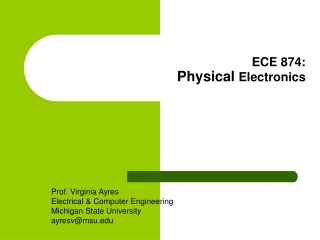DownloadDownload PresentationECE 874: Physical Electronics

# ECE 874: Physical Electronics

Télécharger la présentation## ECE 874: Physical Electronics

- - - - - - - - - - - - - - - - - - - - - - - - - - - E N D - - - - - - - - - - - - - - - - - - - - - - - - - - -
##### Presentation Transcript

1. ECE 874:Physical Electronics Prof. Virginia Ayres Electrical & Computer Engineering Michigan State University ayresv@msu.edu

2. Lecture 14, 16 Oct 12 VM Ayres, ECE874, F12

3. Three different “a”: - lattice constant - “unit cell” of a periodic potential, p.52 - well width I VM Ayres, ECE874, F12

4. I Lattice constant a of the Unit cell GaAs: 5.65 Ang “unit cell” a of a 1-D periodic potential Block theorem Well width a Kronig-Penney model for a 1-D periodic potential VM Ayres, ECE874, F12

5. Example problem: An electron is moving along the  direction in GaAs, lattice constant = 5.65 Ang. (a) Write down both versions of the Block theorem explicitly solving for the “unit cell” of the periodic potential in terms of the lattice constant. (b) Draw a model of the transport environment using the Kronig-Penney model where the well width is 20% of the “unit cell” of a periodic potential. Write the dimensions in terms of the lattice constant. +z +x +y VM Ayres, ECE874, F12

6. Example problem: An electron is moving along the  direction in GaAs, lattice constant = 5.65 Ang.  Face diagonal distance = ✔2 a Distance between atoms = ✔2 a/2 +z +x +y VM Ayres, ECE874, F12

7. VM Ayres, ECE874, F12

8. (b) +z +z +x  +x  +y +y Rotate  to go “straight” VM Ayres, ECE874, F12

9. b + a = aBl = ✔2 aLC/2 = 3.995 Ang (b) b = 0.8 (aBl = 3.995 Ang) = 3.196 Ang aKP = 0.2 (aBl = 3.995 Ang) = 0.799 Ang b aKP  VM Ayres, ECE874, F12

10. Finite Well boundary conditions, Chp. 02: VM Ayres, ECE874, F12

11. Finite Well allowed energy levels, Chp. 02: Graphical solution for number and values of energy levels E1, E2,…in eV. a is the finite well width. VM Ayres, ECE874, F12

12. Similar for Kronig-Penney model but new periodicity requirements: VM Ayres, ECE874, F12

13. Kronig-Penney model allowed energy levels, Chp. 03: Graphical solution for number and values of energy levels E1, E2,…in eV. a = width of well, b = width of barrier, a + b = Block periodicity aBl VM Ayres, ECE874, F12

14. Kronig-Penney model allowed energy levels, Chp. 03: Graphical solution for number and values of energy levels E1, E2,…in eV. Also have values for k from RHS. VM Ayres, ECE874, F12

15. Example problem: (a) What are the allowed (normalized) energies and also the forbidden energy gaps for the 1st-3rd energy bands of the crystal system shown below? (b) What are the corresponding (energy, momentum) values? Take three equally spaced k values from each energy band. VM Ayres, ECE874, F12

16. k = ± p a + b k = 0 0.5 VM Ayres, ECE874, F12

17. (a) VM Ayres, ECE874, F12

18. (b) VM Ayres, ECE874, F12

19. “Reduced zone” representation of allowed E-k states in a 1-D crystal VM Ayres, ECE874, F12

20. k = ± p a + b k = 0 VM Ayres, ECE874, F12